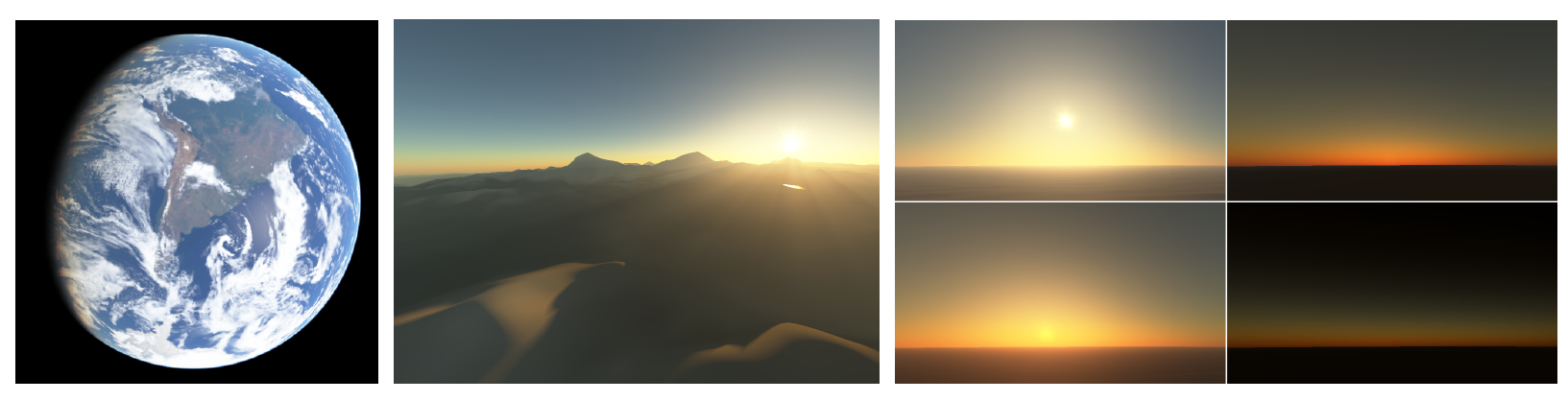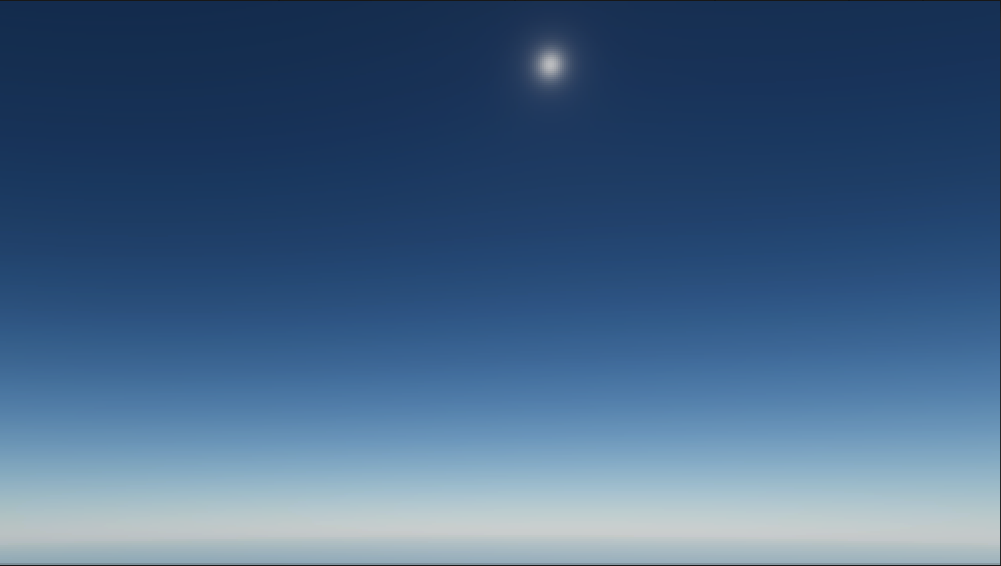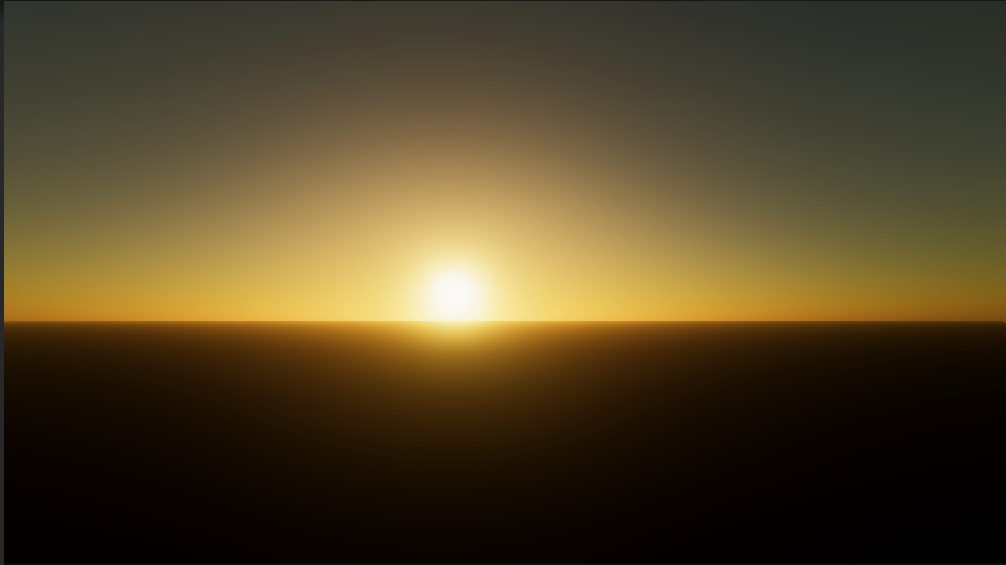0%[BN08]的论文《Precomputed Atmospheric Scattering》表示可在任意位置实时渲染大气散射效果。论文提出了预结算散射效果放入4D纹理中，在着色器中只需要查找表的消耗来得到当前散射结果，从而提高了渲染效率。

##### 计算散射积分

$L_{In} = \int_{C}^{O}L_{Sun}e^{-T(A(s)->P(s))}e^{-T(P(s)->C)} * V(P(s))(\beta_{R}^{s}e^{-h(s)/H_{R}}p_{R}(\theta)+\beta_{M}^{s}e^{-h(s)/H_{M}}p_{M}(\theta)) ds$

* 海拔高度
* 角度：相机方向和太阳方向的夹角。


##### 光学深度计算

$T(A->B) = \beta_{R}^{e}\int_{A}^{B}e^{-h(t)/H_{R}dt} + \beta_{M}^{e}\int_{A}^{B}e^{-h(t)/H_{M}dt}$

$T(A->P) = \beta_{R}^{e}.xyzD[h,\cos{\varphi}].x + \beta_{M}^{e}.xyzD[h,\cos{\varphi}].y$

##### 粒子密度
###### 纹理参数化

1. 任意高度：真实世界的大气高度数值为80000.0f，单位是米。换个思路，用V坐标做插值因子。
2. 任意方向：想象一个圆，任意方向可为单位圆上的一点，因此任意方向可表示为$\cos{varphi}$和$\sin{varphi}$。

##### 渲染结果##### 参考资料
1. GPU Pro 5: High Performance Outdoor Light Scattering Using Epipolar Sampling.
2. Precomputed Atmospheric Scattering